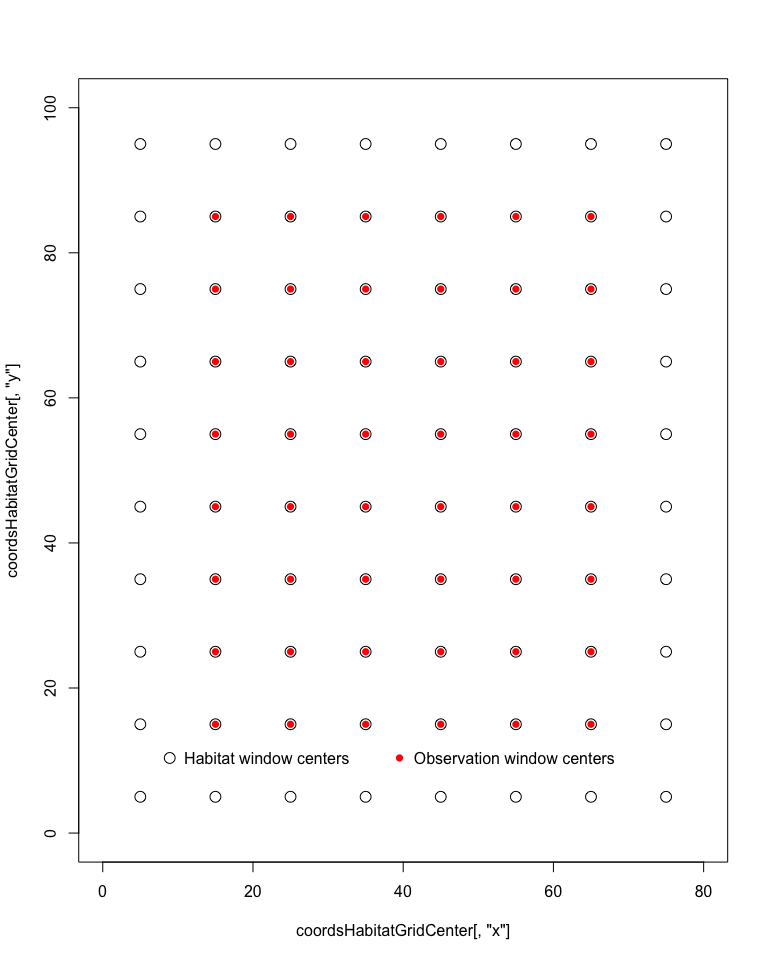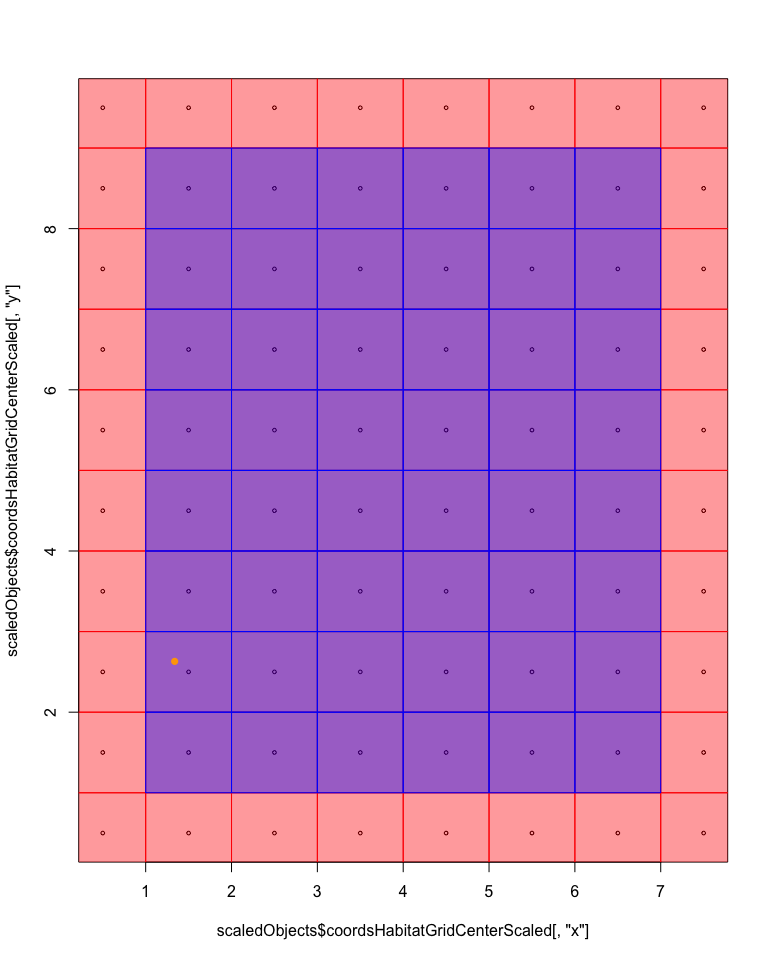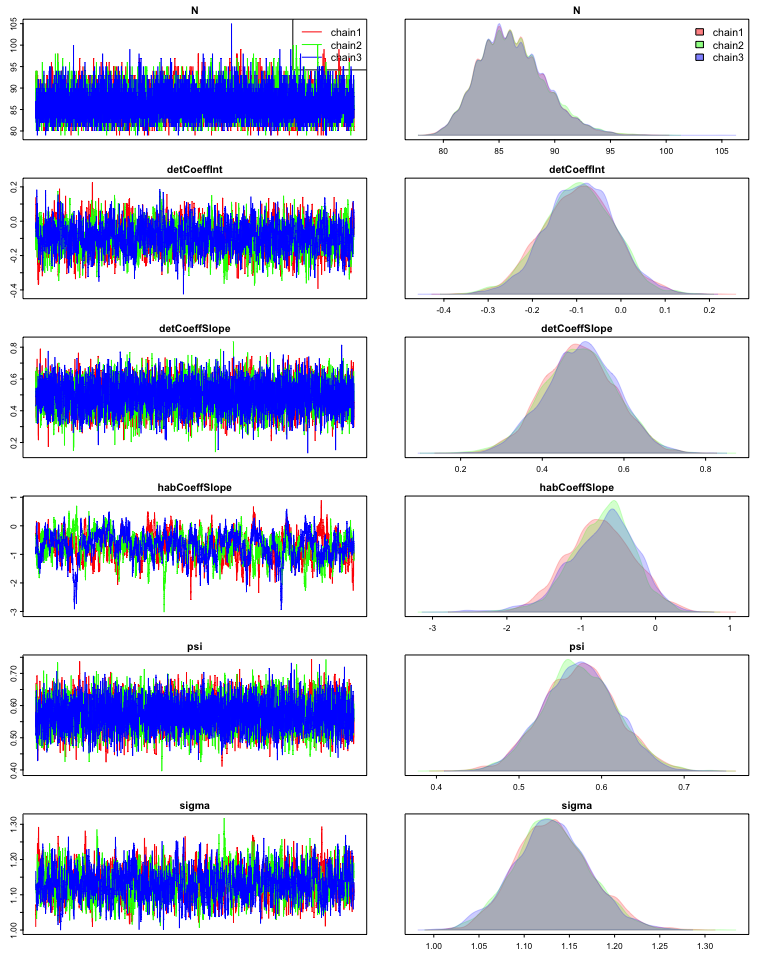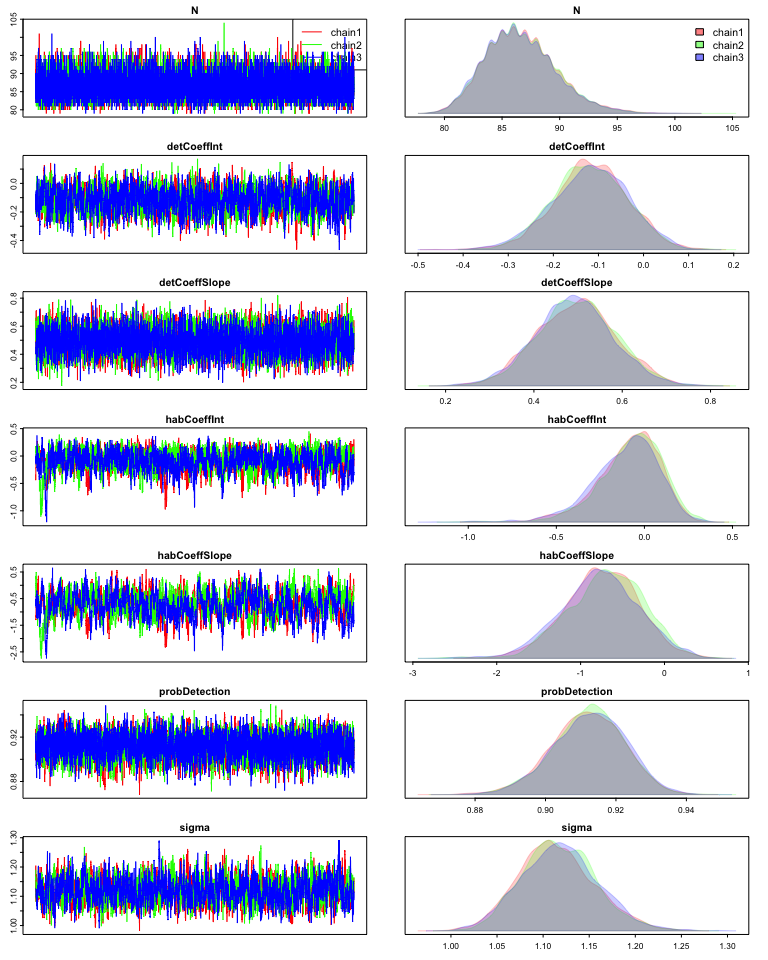# Using nimbleSCR to simulate from and fit Bayesian SCR models

#### 2021-10-25

In this vignette, we demonstrate how to use the nimbleSCR (Bischof et al. 2020) and NIMBLE packages (de Valpine et al. 2017; NIMBLE Development Team 2020) to simulate spatial capture-recapture (SCR) data and fit flexible and efficient Bayesian SCR models via a set of point process functions. Users with real-life SCR data can use this vignette as a guidance for preparing the input data and fitting appropriate Bayesian SCR models in NIMBLE.

## Load packages
library(nimble)
library(nimbleSCR)
library(basicMCMCplots)

## 1. Simulate SCR data

### 1.1 Habitat and trapping grid

As an example, we create a $$80 \times 100$$ habitat grid with a resolution of 10 for each dimension. On the habitat, we center a $$60 \times 80$$ trapping grid with also a resolution of 10 for each dimension, leaving an untrapped perimeter (buffer) with a width of 20 distance units on each side of the grid.

## Create habitat grid
coordsHabitatGridCenter <- cbind(rep(seq(75, 5, by = -10), 10),
sort(rep(seq(5, 100, by = 10), 8)))
colnames(coordsHabitatGridCenter) <- c("x","y")

## Create trap grid
coordsObsCenter <- cbind(rep(seq(15, 65, by = 10), 8),
sort(rep(seq(15, 85, by = 10), 6)))
colnames(coordsObsCenter) <- c("x","y")

## Plot check
plot(coordsHabitatGridCenter[,"y"] ~ coordsHabitatGridCenter[,"x"],
xlim = c(0,80), ylim = c(0,100),
pch = 1, cex = 1.5)
points(coordsObsCenter[,"y"] ~ coordsObsCenter[,"x"], col="red", pch=16 )
par(xpd=TRUE)
legend(x = 7, y = 13,
legend=c("Habitat window centers", "Observation window centers"),
pt.cex = c(1.5,1),
horiz = T,
pch=c(1,16),
col=c("black", "red"),
bty = 'n')### 1.2 Rescale coordinates

To implement the local evaluation approach when fitting the SCR model (see Milleret et al. (2019) and Turek et al. (2021) for further details), we need to rescale the habitat and trapping grid coordinates so that each habitat cell is of dimension $$1 \times 1$$. We also need to identify the lower and upper coordinates of each habitat cell using the ‘getWindowCoords’ function.

## Rescale coordinates
scaledObjects <- scaleCoordsToHabitatGrid(
coordsData = coordsObsCenter,
coordsHabitatGridCenter = coordsHabitatGridCenter)

## Get lower and upper cell coordinates
lowerAndUpperCoords <- getWindowCoords(
scaledHabGridCenter = scaledObjects$coordsHabitatGridCenterScaled, scaledObsGridCenter = scaledObjects$coordsDataScaled,
plot.check = F)

### 1.3 Model definition

modelCode <- nimbleCode({
##---- SPATIAL PROCESS
## Prior for AC distribution parameter
habCoeffSlope ~ dnorm(0, sd = 10)

## Intensity of the AC distribution point process
habIntensity[1:numHabWindows] <- exp(habCoeffSlope * habCovs[1:numHabWindows])
sumHabIntensity <- sum(habIntensity[1:numHabWindows])
logHabIntensity[1:numHabWindows] <- log(habIntensity[1:numHabWindows])
logSumHabIntensity <- log(sumHabIntensity)

## AC distribution
for(i in 1:M){
sxy[i, 1:2] ~ dbernppAC(
lowerCoords = lowerHabCoords[1:numHabWindows, 1:2],
upperCoords = upperHabCoords[1:numHabWindows, 1:2],
logSumIntensity = logSumHabIntensity,
habitatGrid = habitatGrid[1:numGridRows,1:numGridCols],
numGridRows =  numGridRows,
numGridCols = numGridCols
)
}

##---- DEMOGRAPHIC PROCESS
## Prior for data augmentation
psi ~ dunif(0,1)

## Data augmentation
for (i in 1:M){
z[i] ~ dbern(psi)
}

##---- DETECTION PROCESS
## Priors for detection parameters
sigma ~ dunif(0, 50)
detCoeffInt ~ dnorm(0, sd = 10)
detCoeffSlope ~ dnorm(0, sd = 10)

## Intensity of the detection point process
detIntensity[1:numObsWindows] <- exp(detCoeffInt + detCoeffSlope * detCovs[1:numObsWindows])

## Detection process
for (i in 1:M){
y[i, 1:numMaxPoints, 1:3] ~ dpoisppDetection_normal(
lowerCoords = obsLoCoords[1:numObsWindows, 1:2],
upperCoords = obsUpCoords[1:numObsWindows, 1:2],
s = sxy[i, 1:2],
sd = sigma,
baseIntensities = detIntensity[1:numObsWindows],
numMaxPoints = numMaxPoints,
numWindows = numObsWindows,
indicator = z[i]
)
}

##---- DERIVED QUANTITIES
## Number of individuals in the population
N <- sum(z[1:M])
})

### 1.4 Set up parameter values

We set parameter values for the simulation as below.

sigma <- 1
psi <- 0.6
detCoeffInt <- 0.1
detCoeffSlope <- 0.5
habCoeffSlope <- -1.5

We use the data augmentation approach (Royle and Dorazio 2012) to estimate population size N. Thus, we need to choose a value M for the size of the superpopulation (detected + augmented individuals). Here we set M to be 100. The expected total number of individuals that are truly present in the population is M *psi.

M <- 150

When simulating individual detections using the Poisson point process function ‘dpoispp_Detection_normal,’ all the information is stored in y, a 3D array containing i) the number of detections per individual, ii) the x- and y-coordinates of each detection, and iii) the index of the habitat grid cell for each detection (see ?dpoisppDetection_normal for more details):

• ‘y[ ,1,1]’: number of detections for each individual

• ‘y[ ,2:numDetections,1:2]’: x and y coordinates of the detections

• ‘y[ ,2:numDetections,3]’: IDs of the cells (from lowerAndUpperCoords$habitatGrid) in which the detections fall. Cell IDs can be obtained using the ‘getWindowIndex()’ function. Next, we need to provide the maximum number of detections that can be simulated per individual. We set this to be 19 + 1 to account for the fact that the first element of the second dimension of the detection array (y[ ,1,1]) does not contain detection data but the total number of detections for each individual. numMaxPoints <- 19 + 1 In this simulation, we also incorporate spatial covariates on the intensity of the point processes for AC distribution and individual detections. Values of both covariates are generated under a uniform distribution: Unif[-1, 1]. detCovs <- runif(dim(lowerAndUpperCoords$lowerObsCoords),-1,1)
habCovs <- runif(dim(lowerAndUpperCoords$lowerHabCoords),-1,1) ### 1.5 Create data, constants and initial values Here we prepare objects containing data, constants, and initial values that are needed for creating the NIMBLE model below. nimConstants <- list( M = M, numObsWindows = dim(lowerAndUpperCoords$lowerObsCoords),
numMaxPoints = numMaxPoints,
numHabWindows = dim(lowerAndUpperCoords$upperHabCoords), habitatGrid = lowerAndUpperCoords$habitatGrid,
numGridRows = dim(lowerAndUpperCoords$habitatGrid), numGridCols = dim(lowerAndUpperCoords$habitatGrid))

nimData <- list( obsLoCoords = lowerAndUpperCoords$lowerObsCoords, obsUpCoords = lowerAndUpperCoords$upperObsCoords,
lowerHabCoords = lowerAndUpperCoords$lowerHabCoords, upperHabCoords = lowerAndUpperCoords$upperHabCoords,
detCovs = detCovs,
habCovs = habCovs)

In order to simulate directly from the NIMBLE model, we set the true parameter values as initial values. These will be used by the NIMBLE model object to randomly generate SCR data.

nimInits <- list( psi = psi,
sigma = sigma,
detCoeffInt = detCoeffInt,
detCoeffSlope = detCoeffSlope,
habCoeffSlope = habCoeffSlope)

### 1.6 Create NIMBLE model

We can then build the NIMBLE model.

model <- nimbleModel( code = modelCode,
constants = nimConstants,
data = nimData,
inits = nimInits,
check = F,
calculate = F)

### 1.7 Simulate data

In this section, we demonstrate how to simulate data using the NIMBLE model code. Here, we want to simulate individual AC locations (‘sxy’), individual states (‘z’), and observation data (‘y’), based on the values provided as initial values. We first need to identify which nodes in the model need to be simulated, via the ‘getDependencies’ function in NIMBLE. Then, we can generate values for these nodes using the ‘simulate’ function in NIMBLE.

nodesToSim <- model$getDependencies(names(nimInits), self = F) set.seed(1) model$simulate(nodesToSim, includeData = FALSE)

After running the code above, simulated data are stored in the ‘model’ object. For example, we can access the simulated ‘z’ and check the number of individuals that are truly present in the population:

N <- sum(model$z) We have simulated 89 individuals truly present in the population, of which 82 are detected. To check the simulate data, we can also plot the locations of the simulated activity center and detections for a particular individual. i = 7 ## Number of detections for individual i model$y[i,1,1]
##  0
## Plot of the habitat and trap grids
plot( scaledObjects$coordsHabitatGridCenterScaled[,"y"] ~ scaledObjects$coordsHabitatGridCenterScaled[,"x"],
pch = 1, cex = 0.5)
rect( xleft = lowerAndUpperCoords$lowerHabCoords[,1] , ybottom = lowerAndUpperCoords$lowerHabCoords[,2] ,
xright = lowerAndUpperCoords$upperHabCoords[,1], ytop = lowerAndUpperCoords$upperHabCoords[,2],
col = adjustcolor("red", alpha.f = 0.4),
border = "red")

rect( xleft = lowerAndUpperCoords$lowerObsCoords[,1] , ybottom = lowerAndUpperCoords$lowerObsCoords[,2] ,
xright = lowerAndUpperCoords$upperObsCoords[,1], ytop = lowerAndUpperCoords$upperObsCoords[,2],
border = "blue")

## Plot the activity center of individual i
points( model$sxy[i, 2] ~ model$sxy[i, 1],
col = "orange", pch = 16)

## Plot detections of individual i
dets <- model$y[i,2:model$y[i,1,1], ]
points( dets[,2] ~ dets[,1],
col = "green", pch = 16)

par(xpd = TRUE)
legend(x = -1, y = 13,
legend = c("Habitat windows",
"Observation windows",
"Simulated AC",
"Detections"),
pt.cex = c(1,1),
horiz = T,
pch = c(16, 16, 16, 16),
col = c("red", "blue", "orange", "green"),
bty = 'n')## 2. Fit model with data augmentation

### 2.1. Prepare the input data

We have already defined the model above and now need to build the NIMBLE model again using the simulated data ‘y.’ For simplicity, we use the simulated ‘z’ as initial values. When using real-life SCR data you will need to generate initial ‘z’ values for augmented individuals and initial ‘sxy’ values for all individuals.

nimData1 <- nimData
nimData1$y <- model$y
nimInits1 <- nimInits
nimInits1$z <- model$z
nimInits1$sxy <- model$sxy

## Create and compile the NIMBLE model
model <- nimbleModel( code = modelCode,
constants = nimConstants,
data = nimData1,
inits = nimInits1,
check = F,
calculate = F)
cmodel <- compileNimble(model)
## Check the initial log-likelihood
cmodel$calculate() ##  -1378.456 ### 2.2. Run MCMC with NIMBLE Now we can configure and run the MCMC in NIMBLE to fit the model. MCMCconf <- configureMCMC(model = model, monitors = c("N","sigma","psi","detCoeffInt", "detCoeffSlope","habCoeffSlope"), control = list(reflective = TRUE), thin = 1) ## ===== Monitors ===== ## thin = 1: N, detCoeffInt, detCoeffSlope, habCoeffSlope, psi, sigma ## ===== Samplers ===== ## binary sampler (150) ## - z[] (150 elements) ## RW_block sampler (150) ## - sxy[] (150 multivariate elements) ## RW sampler (5) ## - habCoeffSlope ## - psi ## - sigma ## - detCoeffInt ## - detCoeffSlope MCMC <- buildMCMC(MCMCconf) cMCMC <- compileNimble(MCMC, project = model, resetFunctions = TRUE) ## Run MCMC MCMCRuntime <- system.time(samples <- runMCMC( mcmc = cMCMC, nburnin = 500, niter = 10000, nchains = 3, samplesAsCodaMCMC = TRUE)) ## Print runtime MCMCRuntime ## user system elapsed ## 400.58 0.00 400.81 ## Traceplots and density plots for the tracked parameters chainsPlot(samples)## 3. Fit model without data augmentation ### 3.1. Model definition We use the same simulated dataset to demonstrate how to fit a model using the semi-complete data likelihood (SCDL) approach (King et al. 2016). We first need to re-define the model. modelCodeSemiCompleteLikelihood <- nimbleCode({ #----- SPATIAL PROCESS ## Priors habCoeffInt ~ dnorm(0, sd = 10) habCoeffSlope ~ dnorm(0, sd = 10) ## Intensity of the AC distribution point process habIntensity[1:numHabWindows] <- exp(habCoeffInt + habCoeffSlope * habCovs[1:numHabWindows]) sumHabIntensity <- sum(habIntensity[1:numHabWindows]) logHabIntensity[1:numHabWindows] <- log(habIntensity[1:numHabWindows]) logSumHabIntensity <- log(sum(habIntensity[1:numHabWindows] )) ## AC distribution for(i in 1:nDetected){ sxy[i, 1:2] ~ dbernppAC( lowerCoords = lowerHabCoords[1:numHabWindows, 1:2], upperCoords = upperHabCoords[1:numHabWindows, 1:2], logIntensities = logHabIntensity[1:numHabWindows], logSumIntensity = logSumHabIntensity, habitatGrid = habitatGrid[1:numGridRows,1:numGridCols], numGridRows = numGridRows, numGridCols = numGridCols ) } ##---- DEMOGRAPHIC PROCESS ## Number of individuals in the population N ~ dpois(sumHabIntensity) ## Number of detected individuals nDetectedIndiv ~ dbin(probDetection, N) ##---- DETECTION PROCESS ## Probability that an individual in the population is detected at least once ## i.e. 1 - void probability over all detection windows probDetection <- 1 - marginalVoidProbNumIntegration( quadNodes = quadNodes[1:nNodes, 1:2, 1:numHabWindows], quadWeights = quadWeights[1:numHabWindows], numNodes = numNodes[1:numHabWindows], lowerCoords = obsLoCoords[1:numObsWindows, 1:2], upperCoords = obsUpCoords[1:numObsWindows, 1:2], sd = sigma, baseIntensities = detIntensity[1:numObsWindows], habIntensities = habIntensity[1:numHabWindows], sumHabIntensity = sumHabIntensity, numObsWindows = numObsWindows, numHabWindows = numHabWindows ) ## Priors for detection parameters sigma ~ dunif(0, 50) detCoeffInt ~ dnorm(0, sd = 10) detCoeffSlope ~ dnorm(0, sd = 10) ## Intensity of the detection point process detIntensity[1:numObsWindows] <- exp(detCoeffInt + detCoeffSlope * detCovs[1:numObsWindows]) ## Detection process ## Note that this conditions on the fact that individuals are detected (at least once) ## So, at the bottom of this model code we deduct log(probDetection) from the log-likelihood ## function for each individual for (i in 1:nDetected){ y[i, 1:numMaxPoints, 1:3] ~ dpoisppDetection_normal( lowerCoords = obsLoCoords[1:numObsWindows, 1:2], upperCoords = obsUpCoords[1:numObsWindows, 1:2], s = sxy[i, 1:2], sd = sigma, baseIntensities = detIntensity[1:numObsWindows], numMaxPoints = numMaxPoints, numWindows = numObsWindows, indicator = 1 ) } ## Normalization: normData can be any scalar in the data provided when building the model ## The dnormalizer is a custom distribution defined for efficiency, where the input data ## does not matter. It makes it possible to use the general dpoippDetection_normal function ## when either data augmentation or the SCDL is employed logDetProb <- log(probDetection) normData ~ dnormalizer(logNormConstant = -M * logDetProb) }) ### 3.2. Prepare the input data We use the same simulated data as above. Since we do not use data augmentation here, we have to remove all individuals that are not detected from ‘y’ and ‘sxy.’ idDetected <- which(nimData1$y[,1,1] > 0)
## Subset data to detected individuals only
nimData1$y <- nimData1$y[idDetected,,]
## Provide the number of detected individuals as constant
nimConstants$nDetected <- length(idDetected) ## With this model, we also need to provide the number of detected individuals as data for the estimation of population size. nimData1$nDetectedIndiv <- length(idDetected)
## As mentioned above, "normData" can take any value.
nimData1$normData <- 1 ## We also provide initial values for the new parameters that need to be estimated nimInits1$N <- 100
nimInits1$habCoeffInt <- 0.5 nimInits1$sxy <- nimInits1$sxy[idDetected,] The values below are needed to calculate the void probability numerically (i.e. the probability that one individual is detected at least once) using the midpoint rule. ## Number of equal subintervals for each dimension of a grid cell nPtsPerDim <- 2 ## Number of points to use for the numerical integration for each grid cell nNodes <- nPtsPerDim^2 ## Generate midpoint nodes coordinates for numerical integration using the "getMidPointNodes" function nodesRes <- getMidPointNodes( nimData1$lowerHabCoords,
nimData1$upperHabCoords, nPtsPerDim) ## Add this info to the data and constant objects nimData1$quadNodes <- nodesRes$quadNodes nimData1$quadWeights <- nodesRes$quadWeights nimData1$numNodes <- rep(nNodes,dim(nimData1$lowerHabCoords)) nimConstants$nNodes <- dim(nodesRes$quadNodes) ### 3.3. Run MCMC with NIMBLE Finally we can re-build the model and run the MCMC to fit the SCDL model. model <- nimbleModel(code = modelCodeSemiCompleteLikelihood, constants = nimConstants, data = nimData1, inits = nimInits1, check = F, calculate = F) model$calculate()
##  -1029.08
cmodel <- compileNimble(model)
cmodel\$calculate()
##  -1029.08
MCMCconf <- configureMCMC(model = model,
monitors =  c("N","sigma","probDetection","habCoeffInt", "detCoeffInt","detCoeffSlope","habCoeffSlope"),
control = list(reflective = TRUE),
thin = 1)
## ===== Monitors =====
## thin = 1: N, detCoeffInt, detCoeffSlope, habCoeffInt, habCoeffSlope, probDetection, sigma
## ===== Samplers =====
## slice sampler (1)
##   - N
## RW_block sampler (82)
##   - sxy[]  (82 multivariate elements)
## RW sampler (5)
##   - habCoeffInt
##   - habCoeffSlope
##   - sigma
##   - detCoeffInt
##   - detCoeffSlope
MCMC <- buildMCMC(MCMCconf)
cMCMC <- compileNimble(MCMC, project = model, resetFunctions = TRUE)

## Run MCMC
MCMCRuntime1 <- system.time(samples1 <- runMCMC( mcmc = cMCMC,
nburnin = 500,
niter = 10000,
nchains = 3,
samplesAsCodaMCMC = TRUE))
MCMCRuntime1
##    user  system elapsed
## 1663.47    0.01 1664.00
## Plot check
chainsPlot(samples1)